## Magic SquareA (normal) magic square consists of the distinct Positive Integers 1, 2, ...,such that the sum of thenumbers in any horizontal, vertical, or main diagonal line is always the same Magic ConstantThe unique normal square of order three was known to the ancient Chinese, who called it the Lo Shu. A version of the order 4 magic square with the numbers 15 and 14 in adjacent middle columns in the bottom row is called Dürer's Magic Square. Magic squares of order 3 through 8 are shown above.

The Magic Constant for anth order magic square starting with an Integerand with entries in an increasing Arithmetic Series with difference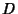between terms is(Hunter and Madachy 1975). If every number in a magic square is subtracted from, another magic square is obtained called the complementary magic square. Squares which are magic under multiplication instead of addition can be constructed and are known as Multiplication Magic Squares. In addition, squares which are magic under both addition and multiplication can be constructed and are known as Addition-Multiplication Magic Squares (Hunter and Madachy 1975).

A square that fails to be magic only because one or both of the main diagonal sums do not equal the Magic Constant is called a Semimagic Square. If all diagonals (including those obtained by wrapping around) of a magic square sum to the Magic Constant, the square is said to be a Panmagic Square (also called a Diabolical Square or Pandiagonal Square). If replacing each number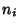by its squareproduces another magic square, the square is said to be a Bimagic Square (or Doubly Magic Square). If a square is magic for,, and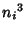, it is called a Trebly Magic Square. If all pairs of numbers symmetrically opposite the center sum to, the square is said to be an Associative Magic Square.Kraitchik (1942) gives general techniques of constructing Even and Odd squares of order. ForOdd, a very straightforward technique known as the Siamese method can be used, as illustrated above (Kraitchik 1942, pp. 148-149). It begins by placing a 1 in any location (in the center square of the top row in the above example), then incrementally placing subsequent numbers in the square one unit above and to the right. The counting is wrapped around, so that falling off the top returns on the bottom and falling off the right returns on the left. When a square is encountered which is already filled, the next number is instead placed below the previous one and the method continues as before. The method, also called de la Loubere's method, is purported to have been first reported in the West when de la Loubere returned to France after serving as ambassador to Siam.

A generalization of this method uses an ordinary vector''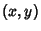which gives the offset for each noncolliding move and a break vector''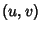which gives the offset to introduce upon a collision. The standard Siamese method therefore has ordinary vector (1,and break vector (0, 1). In order for this to produce a magic square, each break move must end up on an unfilled cell. Special classes of magic squares can be constructed by considering the absolute sums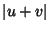,,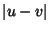, and. Call the set of these numbers the sumdiffs (sums and differences). If all sumdiffs are Relatively Prime toand the square is a magic square, then the square is also a Panmagic Square. This theory originated with de la Hire. The following table gives the sumdiffs for particular choices of ordinary and break vectors.

 Ordinary Vector Break Vector Sumdiffs Magic Squares Panmagic Squares (1,) (0, 1) (1, 3)none (1,) (0, 2) (0, 2)none (2, 1) (1,) (1, 2, 3, 4)none (2, 1) (1,) (0, 1, 2, 3)(2, 1) (1, 0) (0, 1, 2)none (2, 1) (1, 2) (0, 1, 2, 3)noneA second method for generating magic squares of Odd order has been discussed by J. H. Conway under the name of the lozenge'' method. As illustrated above, in this method, the Odd numbers are built up along diagonal lines in the shape of a Diamond in the central part of the square. The Even numbers which were missed are then added sequentially along the continuation of the diagonal obtained by wrapping around the square until the wrapped diagonal reaches its initial point. In the above square, the first diagonal therefore fills in 1, 3, 5, 2, 4, the second diagonal fills in 7, 9, 6, 8, 10, and so on.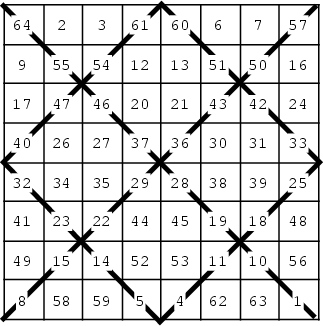An elegant method for constructing magic squares of Doubly Even orderis to draws through eachsubsquare and fill all squares in sequence. Then replace each entryon a crossed-off diagonal by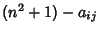or, equivalently, reverse the order of the crossed-out entries. Thus in the above example for, the crossed-out numbers are originally 1, 4, ..., 61, 64, so entry 1 is replaced with 64, 4 with 61, etc.A very elegant method for constructing magic squares of Singly Even orderwith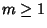(there is no magic square of order 2) is due to J. H. Conway, who calls it the LUX'' method. Create an array consisting ofrows ofs, 1 row of Us, androws of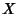s, all of length. Interchange the middle U with the L above it. Now generate the magic square of orderusing the Siamese method centered on the array of letters (starting in the center square of the top row), but fill each set of four squares surrounding a letter sequentially according to the order prescribed by the letter. That order is illustrated on the left side of the above figure, and the completed square is illustrated to the right. The shapes'' of the letters L, U, and X naturally suggest the filling order, hence the name of the algorithm.

It is an unsolved problem to determine the number of magic squares of an arbitrary order, but the number of distinct magic squares (excluding those obtained by rotation and reflection) of order, 2, ... are 1, 0, 1, 880, 275305224, ... (Sloane's A006052; Madachy 1979, p. 87). The 880 squares of order four were enumerated by Frenicle de Bessy in the seventeenth century, and are illustrated in Berlekamp et al. (1982, pp. 778-783). The number of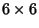squares is not known.The above magic squares consist only of Primes and were discovered by E. Dudeney (1970) and A. W. Johnson, Jr. (Dewdney 1988). Madachy (1979, pp. 93-96) and Rivera discuss other magic squares composed of Primes.Benjamin Franklin constructed the above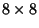Panmagic Square having Magic Constant 260. Any half-row or half-column in this square totals 130, and the four corners plus the middle total 260. In addition, bent diagonals (such as 52-3-5-54-10-57-63-16) also total 260 (Madachy 1979, p. 87).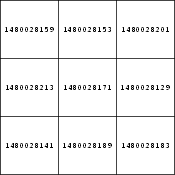In addition to other special types of magic squares, asquare whose entries are consecutive Primes, illustrated above, has been discovered by H. Nelson (Rivera). Variations on magic squares can also be constructed using letters (either in defining the square or as entries in it), such as the Alphamagic Square and Templar Magic Square.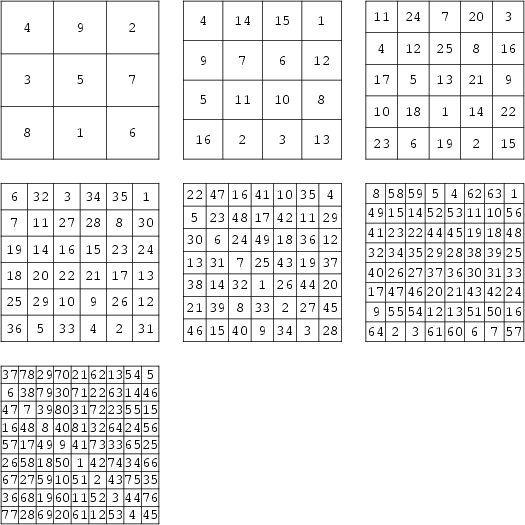Various numerological properties have also been associated with magic squares. Pivari associates the squares illustrated above with Saturn, Jupiter, Mars, the Sun, Venus, Mercury, and the Moon, respectively. Attractive patterns are obtained by connecting consecutive numbers in each of the squares (with the exception of the Sun magic square).

See also Addition-Multiplication Magic Square Alphamagic Square, Antimagic Square, Associative Magic Square, Bimagic Square, Border Square, Dürer's Magic Square, Euler Square, Franklin Magic Square, Gnomon Magic Square, Heterosquare, Latin Square, Magic Circles, Magic Constant, Magic Cube, Magic Hexagon, Magic Labeling, Magic Series, Magic Tour, Multimagic Square, Multiplication Magic Square, Panmagic Square, Semimagic Square, Talisman Square, Templar Magic Square, Trimagic Square

References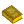Magic Squares

Abe, G. Unsolved Problems on Magic Squares.'' Disc. Math. 127, 3-13, 1994.

Alejandre, S. Suzanne Alejandre's Magic Squares.'' http://forum.swarthmore.edu/alejandre/magic.square.html.

Andrews, W. S. Magic Squares and Cubes, 2nd rev. ed. New York: Dover, 1960.

Ball, W. W. R. and Coxeter, H. S. M. Magic Squares.'' Ch. 7 in Mathematical Recreations and Essays, 13th ed. New York: Dover, 1987.

Barnard, F. A. P. Theory of Magic Squares and Cubes.'' Memoirs Natl. Acad. Sci. 4, 209-270, 1888.

Benson, W. H. and Jacoby, O. New Recreations with Magic Squares. New York: Dover, 1976.

Berlekamp, E. R.; Conway, J. H; and Guy, R. K. Winning Ways, For Your Mathematical Plays, Vol. 2: Games in Particular. London: Academic Press, 1982.

Dewdney, A. K. Computer Recreations: How to Pan for Primes in Numerical Gravel.'' Sci. Amer. 259, pp. 120-123, July 1988.

Dudeney, E. Amusements in Mathematics. New York: Dover, 1970.

Fults, J. L. Magic Squares. Chicago, IL: Open Court, 1974.

Gardner, M. Magic Squares.'' Ch. 12 in The Second Scientific American Book of Mathematical Puzzles & Diversions: A New Selection. New York: Simon and Schuster, 1961.

Gardner, M. Magic Squares and Cubes.'' Ch. 17 in Time Travel and Other Mathematical Bewilderments. New York: W. H. Freeman, 1988.

Grogono, A. W. Magic Squares by Grog.'' http://www.grogono.com/magic/.

Heinz, H. Magic Squares.'' http://www.geocities.com/CapeCanaveral/Launchpad/4057/magicsquare.htm.

Hirayama, A. and Abe, G. Researches in Magic Squares. Osaka, Japan: Osaka Kyoikutosho, 1983.

Horner, J. On the Algebra of Magic Squares, I., II., and III.'' Quart. J. Pure Appl. Math. 11, 57-65, 123-131, and 213-224, 1871.

Hunter, J. A. H. and Madachy, J. S. Mystic Arrays.'' Ch. 3 in Mathematical Diversions. New York: Dover, pp. 23-34, 1975.

Kanada, Y. Magic Square Page.'' http://www.st.rim.or.jp/~kanada/puzzles/magic-square.html.

Kraitchik, M. Magic Squares.'' Ch. 7 in Mathematical Recreations. New York: Norton, pp. 142-192, 1942.

Lei, A. Magic Square, Cube, Hypercube.'' http://www.cs.ust.hk/~philipl/magic/.

Madachy, J. S. Magic and Antimagic Squares.'' Ch. 4 in Madachy's Mathematical Recreations. New York: Dover, pp. 85-113, 1979.

Moran, J. The Wonders of Magic Squares. New York: Vintage, 1982.

Pappas, T. Magic Squares,'' The Special' Magic Square,'' The Pyramid Method for Making Magic Squares,'' Ancient Tibetan Magic Square,'' Magic Line.','' and A Chinese Magic Square.'' The Joy of Mathematics. San Carlos, CA: Wide World Publ./Tetra, pp. 82-87, 112, 133, 169, and 179, 1989.

Pivari, F. Nice Examples.'' http://www.geocities.com/CapeCanaveral/Lab/3469/examples.html.

Pivari, F. Simple Magic Square Checker and GIF Maker.'' http://www.geocities.com/CapeCanaveral/Lab/3469/squaremaker.html.

Rivera, C. Problems & Puzzles (Puzzles): Magic Squares with Consecutive Primes.'' http://www.sci.net.mx/~crivera/puzzles/puzz_003.htm.

Rivera, C. Problems & Puzzles (Puzzles): Prime-Magical Squares.'' http://www.sci.net.mx/~crivera/puzzles/puzz_004.htm.

Sloane, N. J. A. Sequence A006052/M5482 in An On-Line Version of the Encyclopedia of Integer Sequences.'' http://www.research.att.com/~njas/sequences/eisonline.html and Sloane, N. J. A. and Plouffe, S. The Encyclopedia of Integer Sequences. San Diego: Academic Press, 1995.

Suzuki, M. Magic Squares.'' http://www.pse.che.tohoku.ac.jp/~msuzuki/MagicSquare.html.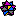Weisstein, E. W. Magic Squares.'' Mathematica notebook MagicSquares.m.